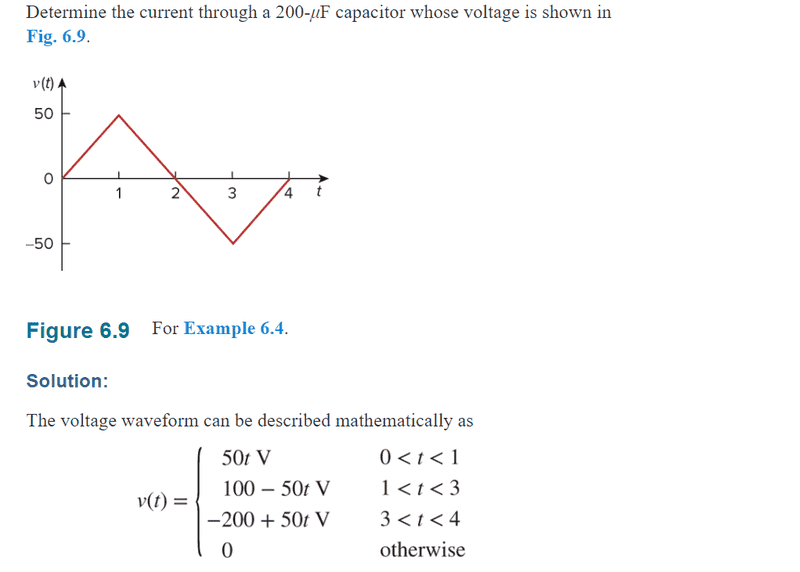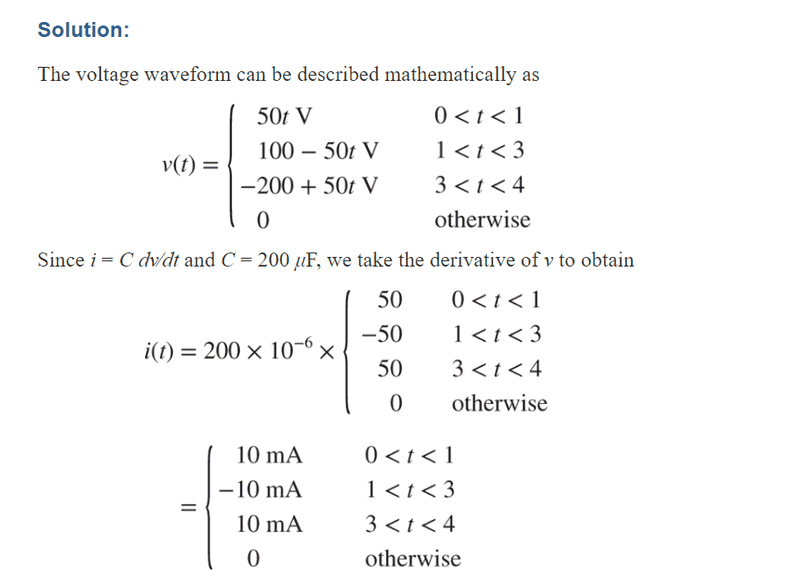# Help Deciphering Voltage-vs-time Graph

• legatobluesummers
In summary, according to these equations, the voltage is found by taking the derivative of the graph, multiplied by the number of Farads given, and then shift the line to match where it is on the graph.f

#### legatobluesummers

Member advised to use the formatting template for all homework help requests
I'm asking how they managed to get those formulas to describe the graph.

a few of my homework questions rely on me being able to get the correct formulas from a graph like this. So i can then find the voltage and amperes in the questions. I'm just trying to understand how
50t represents 0 < t < 1
100 - 50t " " 1 < t < 3
-200 + 50t " " 3 < t < 4

edit: I'm supposed to take the derivatve of v (dv/dt) to get the voltage from the graph and then multiply it by the farads given to get amperes

How do these numbers make sense from the graph? I've thought until I can think no longer.
from 0 to 1, the difference is clearly 50, but why the t variable? 50*1 does equal 50.
from 1 to 3, the point 3 stops at -50, it's vertical magnitude is a negative 100, but it is written as 100-50t,
from 3 to 4, the total magnitude from 0 to 4 is 200, but why is it negative and the 50t positive?

surely there is something stupidly obvious I'm missing here.#### Attachments

Last edited:
from 0 to 1, the difference is clearly 50, but why the t variable? 50*1 does equal 50.

That's right. Plugging 1 in for ##t## gives you 50*1 = 50 V.

from 1 to 3, the point 3 stops at -50, it's vertical magnitude is a negative 100, but it is written as 100-50t,

Plug in 3 to the formula and see what you get.

from 3 to 4, the total magnitude from 0 to 4 is 200, but why is it negative and the 50t positive?

Plug 3 and then 4 into the equation. You'll find that it precisely gives you that part of the graph.

assuming I'm going backwards for difference,
1) 50(1) - 50(0) = 50
2) 50(3) - 50(1) = 150 - 50
3) 50(4) - 50(3) = 200 - 150
i guess i don't know what the formula is

i guess i don't know what the formula is

I'm not sure what you're asking. The formulas for the voltage are given in the picture. Are you asking something else?

I'm asking how they managed to get those formulas to describe the graph.

a few of my homework questions rely on me being able to get the correct formulas from a graph like this. So i can then find the voltage and amperes in the questions. I'm just trying to understand how
50t represents 0 < t < 1
100 - 50t " " 1 < t < 3
-200 + 50t " " 3 < t < 4

edit: I'm supposed to take the derivatve of v (dv/dt) to get the voltage from the graph and then multiply it by the farads given to get amperes#### Attachments

I'm asking how they managed to get those formulas to describe the graph.

Good question. The first thing I would do is find the slope for each part. This one is easy, as each part has a slope of either 50 or -50. From there it's just a matter of adding or subtracting the right numbers to make the equations fit the graph.

For part 1, the line starts at zero, so we don't need an offset at all. It's just 50t.
For part 2, the voltage starts at +50, so we need to take that into account in our formula. The slope is negative, so our ##t## variable will have a -50 in front of it. We have to add a number that makes -50t equal +50 at t=1, 0 at t=2, and -50 at t=3. So let's write a general formula:
##-50t+x=V##

Now write 3 equations using that general formula with the t variable replaced by 1, 2, and 3 and V replaced by 50, 0, and -50.

##-50(1)+x=50 → -50+x=50##
##-50(2)+x=0 → -100+x=0##
##-50(3)+x=-50 → -150+x=-50##

Solve for x:

##-50+x = 50 → x=50+50 → x=100##
##-100+x = 0 → x=0+100 → x=100##
##-150+x = -50 → x=-50+150 → x=100##

So ##x = 100##. Plugging that back into our general equation gives: ##-50t+100 = V##. Rearranging gives: ##100-50t=V##.
Notice that all three equations give us the same value for x. This means that you actually only need to solve the general equation for one value of t, not 3. Once you solve for one equation, you can put the value of x back into the general formula and plug in the other values of t if you want to check that it is correct.

Part 3 can be found using a similar method.

You are a gentleman and a scholar, thank you sir. I would have never figured that out while on tilt.

•Drakkith
Another method which you might find quicker (think exam time pressure) is to once again realize that each is a straight line segment with a given slope and that from functions 101 you can remember that to shift a line horizontally you subtract a constant offset from the "x" variable.

So for the second line segment, to shift where it passes through the t-axis, write:

##v(t) = -50 (t - 2) ## where the ##2## is the constant offset so that,
##v(t) = 100 - 50t##

Same goes for the third one where the offset would be 4 seconds.